Next: Exercises Up: Fundamentals of Quantum Mechanics Previous: Continuous Eigenvalues

Stationary States

An eigenstate of the energy operator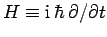corresponding to the eigenvalue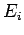satisfies(293)

It is evident that this equation can be solved by writing(294)

whereis a properly normalized stationary (i.e., non-time-varying) wavefunction. The wavefunction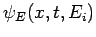corresponds to a so-called stationary state, since the probability densityis non-time-varying. Note that a stationary state is associated with a unique value for the energy. Substitution of the above expression into Schrödinger's equation (137) yields the equation satisfied by the stationary wavefunction: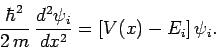(295)

This is known as the time-independent Schrödinger equation. More generally, this equation takes the form(296)

where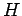is assumed not to be an explicit function of. Of course, thesatisfy the usual orthonormality condition:(297)

Moreover, we can express a general wavefunction as a linear combination of energy eigenstates: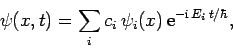(298)

where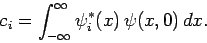(299)

Here,is the probability that a measurement of the energy will yield the eigenvalue. Furthermore, immediately after such a measurement, the system is left in the corresponding energy eigenstate. The generalization of the above results to the case wherehas continuous eigenvalues is straightforward.

If a dynamical variable is represented by some Hermitian operatorwhich commutes with(so that it has simultaneous eigenstates with), and contains no specific time dependence, then it is evident from Eqs. (297) and (298) that the expectation value and variance ofare time independent. In this sense, the dynamical variable in question is a constant of the motion.

SubsectionsNext: Exercises Up: Fundamentals of Quantum Mechanics Previous: Continuous Eigenvalues
Richard Fitzpatrick 2010-07-20